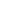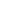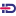SEARCHBROWSEBercu, Bernard, Bigot, Jérémie, Gadat, Sébastienand Siviero, Emilia (2022) A stochastic Gauss-Newton algorithm for regularized semi-discrete optimal transport. Information and Inference: A Journal of the IMA. pp. 1-56.Text Download (150kB)
Identification Number : 10.1093/imaiai/iaac014

## Abstract

We introduce a new second order stochastic algorithm to estimate the entropically regularized optimal transport cost between two probability measures. The source measure can be arbitrary chosen, either absolutely continuous or discrete, while the target measure is assumed to be discrete. To solve the semi-dual formulation of such a regularized and semi-discrete optimal transportation problem, we propose to consider a stochastic Gauss-Newton algorithm that uses a sequence of data sampled from the source measure. This algorithm is shown to be adaptive to the geometry of the underlying convex optimization problem with no important hyperparameter to be accurately tuned. We establish the almost sure convergence and the asymptotic normality of various estimators of interest that are constructed from this stochastic Gauss-Newton algorithm. We also analyze their non-asymptotic rates of convergence for the expected quadratic risk in the absence of strong convexity of the underlying objective function. The results of numerical experiments from simulated data are also reported to illustrate the nite sample properties of this Gauss-Newton algorithm for stochastic
regularized optimal transport, and to show its advantages over the use of the stochastic gradient descent, stochastic Newton and ADAM algorithms.

Item Type: Article English 19 May 2022 Yes Oxford Stochastic optimization, Stochastic Gauss-Newton algorithm, Optimal transport, Entropic regularization, Convergence of random variables. B- ECONOMIE ET FINANCE TSE-R (Toulouse) UT1 09 Sep 2022 13:07 07 Oct 2022 12:44 oai:tse-fr.eu:126866 https://publications.ut-capitole.fr/id/eprint/46211